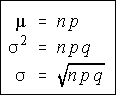# Stats: Binomial Probabilities

## Binomial Experiment

A binomial experiment is an experiment which satisfies these four conditions

• A fixed number of trials
• Each trial is independent of the others
• There are only two outcomes
• The probability of each outcome remains constant from trial to trial.

These can be summarized as: An experiment with a fixed number of independent trials, each of which can only have two possible outcomes.

The fact that each trial is independent actually means that the probabilities remain constant.

#### Examples of binomial experiments

• Tossing a coin 20 times to see how many tails occur.
• Asking 200 people if they watch ABC news.
• Rolling a die to see if a 5 appears.

#### Examples which aren't binomial experiments

• Rolling a die until a 6 appears (not a fixed number of trials)
• Asking 20 people how old they are (not two outcomes)
• Drawing 5 cards from a deck for a poker hand (done without replacement, so not independent)

## Binomial Probability Function

#### Example:

What is the probability of rolling exactly two sixes in 6 rolls of a die?

There are five things you need to do to work a binomial story problem.

1. Define Success first. Success must be for a single trial. Success = "Rolling a 6 on a single die"
2. Define the probability of success (p): p = 1/6
3. Find the probability of failure: q = 5/6
4. Define the number of trials: n = 6
5. Define the number of successes out of those trials: x = 2

Anytime a six appears, it is a success (denoted S) and anytime something else appears, it is a failure (denoted F). The ways you can get exactly 2 successes in 6 trials are given below. The probability of each is written to the right of the way it could occur. Because the trials are independent, the probability of the event (all six dice) is the product of each probability of each outcome (die)

``` 1 FFFFSS  5/6 * 5/6 * 5/6 * 5/6 * 1/6 * 1/6 = (1/6)^2 * (5/6)^4
2 FFFSFS  5/6 * 5/6 * 5/6 * 1/6 * 5/6 * 1/6 = (1/6)^2 * (5/6)^4
3 FFFSSF  5/6 * 5/6 * 5/6 * 1/6 * 1/6 * 5/6 = (1/6)^2 * (5/6)^4
4 FFSFFS  5/6 * 5/6 * 1/6 * 5/6 * 5/6 * 1/6 = (1/6)^2 * (5/6)^4
5 FFSFSF  5/6 * 5/6 * 1/6 * 5/6 * 1/6 * 5/6 = (1/6)^2 * (5/6)^4
6 FFSSFF  5/6 * 5/6 * 1/6 * 1/6 * 5/6 * 5/6 = (1/6)^2 * (5/6)^4
7 FSFFFS  5/6 * 1/6 * 5/6 * 5/6 * 5/6 * 1/6 = (1/6)^2 * (5/6)^4
8 FSFFSF  5/6 * 1/6 * 5/6 * 5/6 * 1/6 * 5/6 = (1/6)^2 * (5/6)^4
9 FSFSFF  5/6 * 1/6 * 5/6 * 1/6 * 5/6 * 5/6 = (1/6)^2 * (5/6)^4
10 FSSFFF  5/6 * 1/6 * 1/6 * 5/6 * 5/6 * 5/6 = (1/6)^2 * (5/6)^4
11 SFFFFS  1/6 * 5/6 * 5/6 * 5/6 * 5/6 * 1/6 = (1/6)^2 * (5/6)^4
12 SFFFSF  1/6 * 5/6 * 5/6 * 5/6 * 1/6 * 5/6 = (1/6)^2 * (5/6)^4
13 SFFSFF  1/6 * 5/6 * 5/6 * 1/6 * 5/6 * 5/6 = (1/6)^2 * (5/6)^4
14 SFSFFF  1/6 * 5/6 * 1/6 * 5/6 * 5/6 * 5/6 = (1/6)^2 * (5/6)^4
15 SSFFFF  1/6 * 1/6 * 5/6 * 5/6 * 5/6 * 5/6 = (1/6)^2 * (5/6)^4
```

Notice that each of the 15 probabilities are exactly the same: (1/6)^2 * (5/6)^4.

Also, note that the 1/6 is the probability of success and you needed 2 successes. The 5/6 is the probability of failure, and if 2 of the 6 trials were success, then 4 of the 6 must be failures. Note that 2 is the value of x and 4 is the value of n-x.

Further note that there are fifteen ways this can occur. This is the number of ways 2 successes can be occur in 6 trials without repetition and order not being important, or a combination of 6 things, 2 at a time.

The probability of getting exactly x success in n trials, with the probability of success on a single trial being p is:

```   P(X=x) = nCx * p^x * q^(n-x)
```

#### Example:

A coin is tossed 10 times. What is the probability that exactly 6 heads will occur.

1. Success = "A head is flipped on a single coin"
2. p = 0.5
3. q = 0.5
4. n = 10
5. x = 6

P(x=6) = 10C6 * 0.5^6 * 0.5^4 = 210 * 0.015625 * 0.0625 = 0.205078125

## Mean, Variance, and Standard Deviation

The mean, variance, and standard deviation of a binomial distribution are extremely easy to find.Another way to remember the variance is mu-q (since the np is mu).

#### Example:

Find the mean, variance, and standard deviation for the number of sixes that appear when rolling 30 dice.

Success = "a six is rolled on a single die". p = 1/6, q = 5/6.

The mean is 30 * (1/6) = 5. The variance is 30 * (1/6) * (5/6) = 25/6. The standard deviation is the square root of the variance = 2.041241452 (approx)